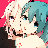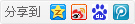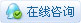##帐号 自动登录 找回密码 密码 立即注册→加入我们
 搜索 热搜: 下载 VB C 实现 编写

# 【C】计算排列组合7主题 261积分

UID
6266

5

37 点

121 个

30 次

0 份

5 小时

2020-9-26发表于 2020-11-2 21:48:19 | 显示全部楼层 |阅读模式

### 欢迎访问技术宅的结界，请注册或者登录吧。

x

1.计算排列：
以下代码按字典序计算1、2、3的全排列：
[C] 纯文本查看 复制代码
#include <stdio.h>
#include <string.h>
#include "StoneValley-master/src/svstring.h"

int cbftvs(void * pitem, size_t param)
{
printf(" %c", *(char *)pitem); // 打印数组中的每个元素
return CBF_CONTINUE;
}

int cbfcmp(const void * px, const void * py)
{
return *(char *)px - *(char *)py;
}

int main(void)
{
auto char c;
ARRAY_Z arrz; // 使用一个数组
strInitArrayZ(&arrz, 3, sizeof(char)); // 初始化数组
memcpy(arrz.pdata, "123", 3); // 将数组设置为1、2、3
do
{
strTraverseArrayZ(&arrz, sizeof(char), cbftvs, 0, FALSE); // 打印数组
printf("\n");
} while (strPermuteArrayZ(&arrz, &c, sizeof(char), cbfcmp, TRUE)); // 按字典序对数组进行排列
strFreeArrayZ(&arrz); // 释放数组
return 0;
}

计算结果：
1 2 3
1 3 2
2 1 3
2 3 1
3 1 2
3 2 1
2.计算组合：
以下代码按字典序计算1、2、3、4中任意取出3个的组合：
[C] 纯文本查看 复制代码
#include <stdio.h>
#include <string.h>
#include "StoneValley-master/src/svstring.h"

int cbftvs(void * pitem, size_t param)
{
printf(" %c", *(char *)pitem); // 打印数组中的每个元素
return CBF_CONTINUE;
}

int cbfcmp(const void * px, const void * py)
{
return *(char *)px - *(char *)py;
}

int main(void)
{
auto char c;
ARRAY_Z arrz, arrb; // 使用数组
strInitArrayZ(&arrz, 3, sizeof(char)); // 初始化数组
strInitArrayZ(&arrb, 4, sizeof(char));
memcpy(arrz.pdata, "123", 3); // 将数组设置为1、2、3就是字典序的第一个组合
memcpy(arrb.pdata, "1234", 4); // 将数组设置为1、2、3、4
do
{
strTraverseArrayZ(&arrz, sizeof(char), cbftvs, 0, FALSE); // 打印数组
printf("\n");
} while (strCombineNextArrayZ(&arrz, &arrb, sizeof(char), cbfcmp)); // 按字典序计算arrb数组的组合
strFreeArrayZ(&arrz); // 释放数组
strFreeArrayZ(&arrb);
return 0;
}


计算结果：
1 2 3
1 2 4
1 3 4
2 3 4

[C] 纯文本查看 复制代码
#include <stdio.h>
#include <string.h>
#include "..\\src\\svstring.h"

#define N_ 4
#define R_ 3
#define strize(a) # a
#define numerize(s) strize(s)
#define paste(a, b, c) a ## b ## c

typedef char MYTYPE;

// Function: cbfcmp
// Desc:     Compare values for MYTYPE values.
// Param:    px: a pointer to a MYTYPE value. py: a pointer to another MYTYPE value.
// Return:   Either 1, -1 or 0 depends on comparison result.
int cbfcmp(const void * px, const void * py)
{
if (*(MYTYPE *)px > *(MYTYPE *)py) return  1;
if (*(MYTYPE *)px < *(MYTYPE *)py) return -1;
return 0;
}

// Function: PrintArrayZ
// Desc:     Print data for an sized array from index 0 to (n - 1).
// Param:    parrz:  pointer to a sized array.
//           n:      Number of items you want to print.
//           size:   Size of each element in the array.
//           bchar:  TRUE: print char; FALSE: print number.
// Return:   N/A.
void PrintArrayZ(P_ARRAY_Z parrz, size_t size, BOOL bchar)
{
char c;
size_t i;
/* Hide a private member by using a macro or a function is not elegant,
* Therefore we cannot use classes in C, this is a better way to circumvent
* altering parrz->num, so that strLevelArrayZ might not be an appendix.
*/
for (i = 0; i < strLevelArrayZ(parrz); ++i)
printf("%c", (c = *(MYTYPE *)(parrz->pdata + i * size), bchar ? c : c - '0'));
printf("\n");
}

// Function: main
// Desc:     Program entry.
// Param:    N/A.
// Return:   0: no error; 1, 2: allocation failure.
int main(void)
{
char q;
int i = 0;
ARRAY_Z n, r;
char pstr[] = "abcd", t;
/* Initialize two arrays. */
if (NULL == strInitArrayZ(&n, N_, sizeof(MYTYPE)))
return 1; /* Allocation failure. */
if (NULL == strInitArrayZ(&r, R_, sizeof(MYTYPE)))
{	/* Another alocation failure. */
i = 2;
}
/* Initialize two arrays. */
memcpy(n.pdata, pstr, N_ * sizeof(MYTYPE));
memcpy(r.pdata, pstr, R_ * sizeof(MYTYPE));
do
fflush(stdin), printf("Would you like to print the result numerically(Y/n)? "), scanf("%c", &q);
while (q != 'Y' && q != 'y' && q != 'N' && q != 'n');
q = !(q & 1);
printf("P(%d, %d) =\n", N_, R_);
do
{	/* Generate all permutations of the current subset for combination. */
while
(	/* Some versions of GCCs would mis-parse the following sentence while VC won't. */
printf(paste("%", numerize(N_), "d:\t"), ++i),
PrintArrayZ(&r, sizeof(MYTYPE), (BOOL)q),
strPermuteArrayZ(&r, &t, sizeof(MYTYPE), cbfcmp, TRUE)
);
}	/* Generate (nCr) circularly. */
while (strCombineNextArrayZ(&r, &n, sizeof(MYTYPE), cbfcmp));
i = 0;
strFreeArrayZ(&r);
strFreeArrayZ(&n);
return i;
}


运行结果如下：
Would you like to print the result numerically(Y/n)? n
P(4, 3) =
1:   abc
2:   acb
3:   bac
4:   bca
5:   cab
6:   cba
7:   abd
10:   bda
11:   dab
12:   dba
13:   acd
16:   cda
17:   dac
18:   dca
19:   bcd
20:   bdc
21:   cbd
22:   cdb
23:   dbc
24:   dcb

[C] 纯文本查看 复制代码
/* Function name: strPermuteArrayZ
* Description:   Permute a fixed size array in dictionary order.
* Parameters:
*      parrz Pointer to a sized array.
*      ptemp Pointer to a buffer whose size equals to each size of the element in the array.
*       size Size of each element in the array.
*     cbfcmp Pointer to a function that compares any two elements in array.
*      bnext Input TRUE to permute an array next; Input FALSE to permute an array previously.
* Return value:  TRUE indicates permutation continued; FALSE indicates permutation ended.
* Caution:       Address of parrz Must Be Allocated first.
*                Users shall manage the buffer that ptemp points at.
*                (*) The size of the buffer of ptemp pointed shall equal to parameter size.
*                (*) Each element in the array shall be unique.
* Tip:           Users may call strUniqueArrayZ to generate a suitable array for permuting.
*                This function references to two similar templates in STL of C Plus Plus.
*/
BOOL strPermuteArrayZ(P_ARRAY_Z parrz, void * ptemp, size_t size, CBF_COMPARE cbfcmp, BOOL bnext)
{
if (strLevelArrayZ(parrz) > 1 && size > 0) /* Worth permuting. */
{	/* ptrl Always points the last element. */
REGISTER PUCHAR ptrl = parrz->pdata + (strLevelArrayZ(parrz) - 1) * size;
REGISTER PUCHAR ptri, ptrj;
for (ptri = ptrl - size, ptrj = ptrl;; ptri -= size, ptrj -= size)
{
REGISTER int r = cbfcmp(ptri, ptrj);
if (bnext ? r < 0 : r > 0)
{
REGISTER PUCHAR ptrk;
for
(
ptrk = ptrl;
(r = cbfcmp(ptrk, ptri)),
!(bnext ? r > 0 : r < 0);
ptrk -= size
);
/* Swap (*i) and (*k). */
svSwap(ptri, ptrk, ptemp, size);
{	/* Reverse array from j to last. */
ARRAY_Z arrt; /* Auxiliary array header for reversing. */
arrt.num   = (size_t)((ptrl - ptrj) / size + 1);
arrt.pdata = ptrj;
strReverseArrayZ(&arrt, ptemp, size);
}
return TRUE;
}
if (ptri <= parrz->pdata)
{	/* Reverse array from first to last. */
strReverseArrayZ(parrz, ptemp, size);
goto Lbl_End_Permuting;
}
}
}
Lbl_End_Permuting:
return FALSE;
}

/* Function name: strCombineNextArrayZ
* Description:   Generate the next combination of an array in dictionary order.
*                If n equaled (parrzn->num) and r equaled (parrzr->num), this function would generate
*                the subset r of parrzn aka (n C r) aka C(n, r) and finally copy the result into parrzr.
* Parameters:
*     parrzr Pointer to an initialized array that contains a result of a previous combination.
*     parrzn Pointer to a sized array that is sorted in increasing order.
*       size Size of each element in both two arrays.
*     cbfcmp Pointer to a function that compares any two elements in two arrays.
* Return value:  TRUE indicates combination continued; FALSE indicates combination ended.
* Caution:       Address of Both parrzn and parrzr Must Be Allocated first.
*                (*) Each element in parrzn shall be unique.
*                (*) Elements in array that parrzn and parrzr pointed shall be sorted in increasing order.
* Tip:           Users may call strUniqueArrayZ(parrzn, ptemp, size, cbfcmp, TRUE);
*                to generate a suitable array for combination.
*/
BOOL strCombineNextArrayZ(P_ARRAY_Z parrzr, P_ARRAY_Z parrzn, size_t size, CBF_COMPARE cbfcmp)
{	/* Assume that the array that parrzn contains has been assigned and sorted yet. */
if (parrzr->num > 0 && parrzr->num < parrzn->num)
{
REGISTER size_t i, j = parrzr->num - 1;
REGISTER PUCHAR pa = &parrzr->pdata[size * j];
REGISTER PUCHAR pt = &parrzn->pdata[size * (parrzn->num - 1)];
/* Compare back through parrzn with parrzr to find a position as pa. */
for (i = 0; i < j; ++i, pt -= size, pa -= size)
if (0 != cbfcmp(pt, pa))
break;
if (0 == cbfcmp(pt, pa))
goto Lbl_End_Combination; /* Combination reaches at the end. */
if (NULL == (pt = (PUCHAR) svBinarySearch(pa, parrzn->pdata, parrzn->num, size, cbfcmp)))
goto Lbl_End_Combination; /* An element in parrzr doesn't match any element in parrzn. */
/* Fill subset r with values in parrzn. */
pt += size;
i = parrzr->num - (pa - parrzr->pdata + size) / size;
do
{
memcpy(pa, pt, size);
pa += size;
pt += size;
}
while (0 != i--);
return TRUE;
}
Lbl_End_Combination:
return FALSE; /* No next combination. */
}

### 评分0xAA55 + 5 + 10 + 10 StoneValley库，我第一次听说

### 本帖被以下淘专辑推荐:本版积分规则 回帖并转播 回帖后跳转到最后一页|申请友链||Archiver|手机版|小黑屋|技术宅的结界 ( 滇ICP备16008837号 )|网站地图

GMT+8, 2020-11-28 04:47 , Processed in 0.097656 second(s), 32 queries , Gzip On.